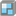• # Ground for the house

## Question related to missionGround for the House

I would like to have some feedback ... couldn't figure out why it's not working.

HTTP_USER_AGENT:

```Mozilla/5.0 (Windows NT 10.0; Win64; x64; rv:64.0) Gecko/20100101 Firefox/64.0

import re

def house(plan):
#replace this for solution
count = 0
flag = 0
flag1 = 0
flag2 = 0
flag3 = 0
flag4 = 0
x = []
y = []
list = plan.split('\n')
print(list)
for i in list:   #collect a substring
# print('first loop')
count += 1
if re.search('#',str(i))!= None:
flag2 = 1
y.append(flag2)
else:
flag2 = 0
y.append(flag2)
for j in i:    #iterate over the substring
# print('second loop')

if j=='#':
print('found #')
flag = 1    #starting point of the house in the line x
r_in = i.rindex('#') + 1    #ending point on the line x

if flag==1:
for k in range(r_in):
flag1 += 1
x.append(flag1)        # x line length
flag1 = 0
break

for l in y:
if l==1:
break
flag3 += 1

for m in reversed(y):
if m==1:
break
flag4 += 1
print('x')
print(x)
print('y')
print(y)
try:
a = max(x)
except ValueError:
a = 0
print('count='+str(count))
print('flag3='+str(flag3))
print('flag4='+str(flag4))
b = count - flag3 - flag4
print('a='+str(a))
print('b='+str(b))
print('area='+str(a*b))
return a*b

if __name__ == '__main__':
print("Example:")
print(house('''
0000000
##00##0
######0
##00##0
#0000#0
'''))

#These "asserts" using only for self-checking and not necessary for auto-testing
assert house('''
0000000
##00##0
######0
##00##0
#0000#0
''') == 24

assert house('''0000000000
#000##000#
##########
##000000##
0000000000
''') == 30

assert house('''0000
0000
#000
''') == 1

assert house('''0000
0000
''') == 0

assert house('''
0##0
0000
#00#
''') == 12

assert house('''
0000000000
000###0000
000#######
000###0000
''') == 21

print("Coding complete? Click 'Check' to earn cool rewards!")
```4